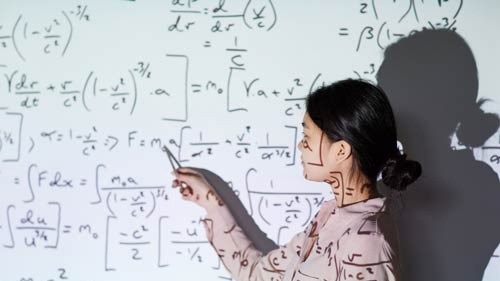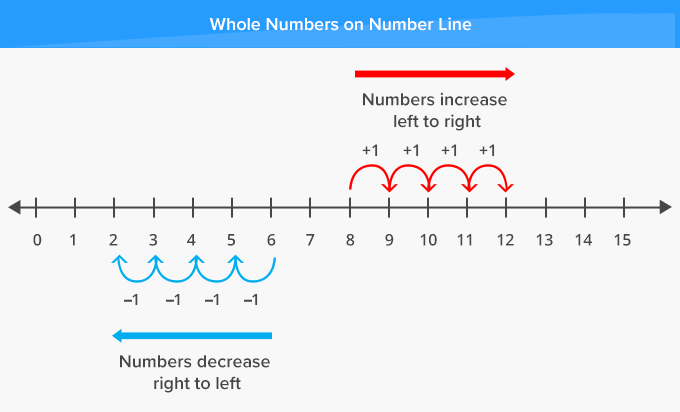By Sean AlexanderStudents make the same algebra mistakes over and over again.  As math tutors, we correct these mistakes constantly. Here is a list of the top 5 most common algebra mistakes and how to fix them forever.  An algebra student who studies these common errors will benefit greatly.

## Algebra Mistake #1

### Not dividing the entire side of the equation

What you do to one side of the equation, you do to the other.  This is one of the most important rules in math.  The most common algebra mistake is not dividing the ENTIRE side of the equation.  Let's look at an example:

$3x+2=6$

$\frac{3x}{3}+2=\frac{6}{3}$

Can you see the mistake?  The student meant to divide both sides of the equation by 3.  On the left-hand side, only the 3x was divided by 3, not the +2.  Therefore the student did not divide the ENTIRE side by 3.  This is the most common algebra mistake.  Here it is done correctly:

$\frac{3x+2}{3}=\frac{6}{3}$

$\frac{3x}{3}+\frac{2}{3}=2$

$x+\frac{2}{3}=2$

$x=\frac{4}{3}$

## Algebra mistake #2

### Not distributing properly using F.O.I.L.

All math tutors have had to correct this mistake a million times. When you have two or more terms raised to a power, you must multiply every combination possible.  For example

$(x+2)^2$

Many students make the following algebra mistake:

$(x+2)^2=x^2+y^2$

This leaves out the cross terms.  Here it is done correctly:

$(x+2)^2=(x+2)(x+2)=x^2+2x+2x+4$

$=x^2+4x+4$

## Algebra Mistake #3

### Confusing the rules for adding and subtracting negative numbers with the rules for multiplying and dividing negative numbers

There are two sets of rules for negative numbers.

1. Adding and subtracting negative numbers
2. Multiplying and dividing negative numbers

Students frequently make the algebra mistake of confusing these two sets of rules.  They use the multiplication rules for addition and vice versa.  Let's take a look at an example mistake

$-3-5=8$

Here the student saw the two minus signs and made the answer positive.  This is the rule for multiplying negative numbers, not addition/subtraction of negative numbers.

$-3-5=-8$

For addition and subtraction of negative numbers, you must think about the number line.A minus sign means move left.  A plus sign means move right.  So in our case, we move left 3 spaces from 0, landing on -3.  We then move an additional 5 spaces to the left, landing on -8.  Let's look at one more example

$3-5=-2$

In this case, we move 3 spaces to the right, landing on +3.  We then move 5 spaces to the left, landing on -2.

For multiplication, there are two rules to learn

1. $(-)*(-)=+$
2. $(-)*(+)=-$

If you multiply two identical signs (two minus's or two plus's) you get a positive number.  If you multiply two opposite signs, you get a negative number.

## Algebra Mistake #4

### Multiplying exponents with the base

Algebra students love to multiply exponents like this

$3^4=12$

This is a common algebra mistake.  An exponent is shorthand for a bunch of multiplication.  In our example

$3^4=(3)*(3)*(3)*(3)=81$

It's important to know where rules come from.  The student that multiplies the base and exponent does not understand what an exponent does.  This is a major gap in the student's comprehension of algebra.

## Algebra Mistake #5

### Turning negative exponents into negative coefficients

Negative exponents do not mean negative coefficients!  Many algebra students don't understand what a negative exponent is.  Here is the mistake

$3^{-2}=-6$

A negative exponent implies the inverse.  In fact

$3^{-2}=\frac{1}{3^2}$

## Conclusion

Algebra mistakes are super common.   Everyone makes them, even your teachers!  The key is to learn how to catch your mistakes.  Preventing the 5 algebra mistakes above will make high school math a breeze.

In addition, you should take great notes in class.  You will need to refer back to them often.  Finally, study hard!  There is no substitute for regular practice.  Take the time to do many practice algebra problems and you'll do great.   Good luck!

Algebra Mistakes FAQ

The best thing you can do to avoid algebra mistakes is to have a system for checking your work.  Once you write down the answer you're not done!  You need to go through the problem line by line as if you were the teacher grading someone else's homework.

If possible, plug your answer back into the original equation and see if you get the same number on each side of the equals sign.  If so, you did it right.  If not, you made an algebra mistake.

Neatness is very important for avoiding algebra mistakes.  Make sure to write as neatly as possible and have everything line up properly.  Graph paper is a wonderful tool for this.

You should only do one mathematical operation per line.  Also, carry everything from the line above to the line below, even if you are not working on it this particular step.  It's common for students to leave out a piece of the equation and then never put it back.  While it can seem like this takes a lot of extra time, the reality is it saves you a ton of algebra mistakes, and thus time.

Don't do messy side calculations.  Many students will do a minor calculation off to the side, this often leads to algebra mistakes.  Make all calculations in line with the rest of your work.

An example of a basic algebra equation is 3x + 5 = 11.

The "x" is a number that you don't know yet.  The goal of algebra is to figure out what the number x is.

The most important rule in basic algebra is "what you do to one side of the equation, you must do to the other."  The goal is to isolate the x by performing equal operations to both sides of the equation.

In the case of our basic algebra equation example 3x + 5 = 11, you would first subtract 5 from both sides to get 3x = 6.

Then you would divide both sides by 3 to get x = 2.  That's how you solve for x in a basic algebra equation!

The 5 most common algebra mistakes are:

1. Not dividing the entire side of the equation.  Instead, the student divides a portion of one side of the equation.

2.  Not distributing properly using F.O.I.L.  The common mistake is to leave out the cross terms.

3.  Mixing the rules for adding and subtracting negative numbers with the rules for multiplying and dividing negative numbers.

4.  Multiplying an exponent by the base.

5.  Turning negative exponents into negative coefficients.

## Author

•COMMAND PILOT, OWNER Sean has been a professional educator for 15 years and has taught math, physics, and astronomy at all levels.  His experience ranges from working at a high school for severe learning differences to teaching advanced physics at Stanford.  After completing his graduate work in theoretical physics Sean founded Alexander Tutoring, with the mission of revealing the deep connections between math and nature to as many students as possible.